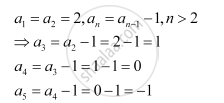# Write the First Five Terms of the Following Sequence and Obtain the Corresponding Series: A_1 = A_2 = 2, A_N = A_(N-1) -1, N > 2 - Mathematics

Write the first five terms of the following sequence and obtain the corresponding series:

a_1 = a_2 = 2, a_n = a_(n-1) -1, n > 2

#### SolutionHence, the first five terms of the sequence are 2, 2, 1, 0, and –1.

The corresponding series is 2 + 2 + 1 + 0 + (–1) + …

Concept: Concept of Series
Is there an error in this question or solution?

#### APPEARS IN

NCERT Class 11 Mathematics
Chapter 9 Sequences and Series
Q 13 | Page 181